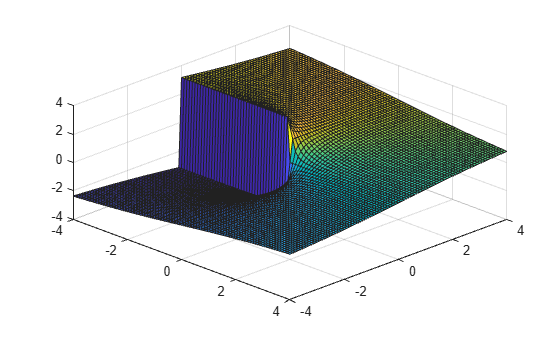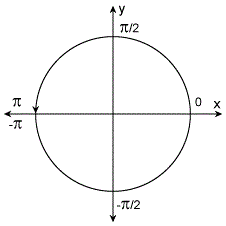# atan2

## 语法

``P = atan2(Y,X)``

## 说明

``P = atan2(Y,X)` 返回 `Y` 和 `X` 的四象限反正切 (tan-1)，该值必须为实数。`atan2` 函数遵循当 `x` 在数学上为零（或者为 `0` 或 `-0`）时 `atan2(x,x)` 返回 `0` 的约定。`

## 示例

`atan2(4,-3)`
```ans = 2.2143 ```

`4 + 3i` 转换为极坐标。

```z = 4 + 3i; r = abs(z)```
```r = 5 ```
`theta = atan2(imag(z),real(z))`
```theta = 0.6435 ```

`theta = angle(z)`
```theta = 0.6435 ```

`r``theta` 转换回原始复数。

`z = r*exp(i*theta)`
```z = 4.0000 + 3.0000i ```

`[X,Y] = meshgrid(-4:0.1:4,-4:0.1:4);`

`P = atan2(Y,X);`

```surf(X,Y,P); view(45,45);```## 输入参数

y 坐标，指定为标量、向量、矩阵、多维数组、表或时间表。输入 `Y``X` 必须具有相同的大小或具有兼容的大小（例如，`Y` 是一个 `M`×`N` 矩阵，`X` 是标量或 `1`×`N` 行向量）。有关详细信息，请参阅基本运算的兼容数组大小

x 坐标，指定为标量、向量、矩阵、多维数组、表或时间表。输入 `Y``X` 必须具有相同的大小或具有兼容的大小（例如，`Y` 是一个 `M`×`N` 矩阵，`X` 是标量或 `1`×`N` 行向量）。有关详细信息，请参阅基本运算的兼容数组大小

## 详细信息

### 四象限反正切### IEEE 合规性

MATLAB® IEEE
`atan2(0,-0)`

`0`

`pi`

`atan2(-0,-0)`

`0`

`-pi`